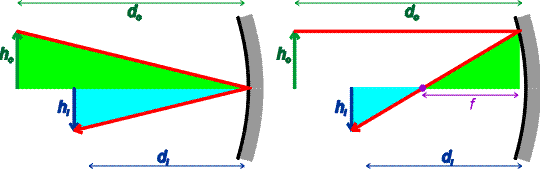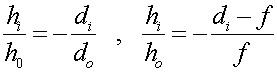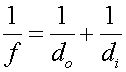Real images On the previous page, we assumed that the light rays approaching the mirror were all parallel. This is true for a distant object, but not true for an object a finite distance removed from the mirror. We now derive the point at which the light is collected, the image distance, as a function of the distance the object is separated from the mirror, the object distance. Consider an object of height ho and an image of height hi below. The two triangles on the left and right are similar since they have the same angles. The two triangles on the right are also similar.The similarity of the triangles allows one to writeOne can combine these two equations, eliminating the ratio of the object and image heights, to obtain a relationship between the distances and focal length.This is a remarkably useful formula. The same formula will be used for both concave (above) and convex mirrors as well as lenses.